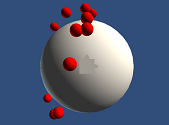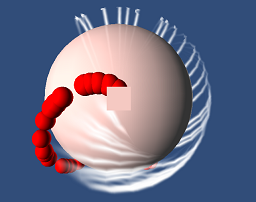# How can I make movement on a sphere?

Hello. I want to make a game like Super Stardust HD.Super Stardust HD

The game takes place on a large sphere that the player travels around. But the trick is, the player doesnt actually move, they spaceship is always in the same place, everything else just rotates. I have scripts for things like the movement, firing, etc. but I cannot figure out how to create the illusion of moving around the sphere (currently the movement is just on a 2D plane like geometry wars, etc., and it cannot be on a sphere for obvious physics reasons).

How would I create (or at least create the illusion of) a sphere that the player can move around?

Thanks.

I thought it was a fun thing to attempt to create from scratch. Here’s my stab at it.

In my scene, I set up a sphere and a cube such that the cube is parented to the sphere and that the cube has 0.1 scale. Then I apply the script to the cube. Now you can control it running along the sphere.

It works like having a 2d coordinate system but instead of dealing with vectors, we’re dealing with quaternions. I use a float angle to determine which way the player is facing on the sphere and then rotate the player around the midpoint of the planet in the direction expressed by cos angle and sin angle. Sideways movement is achieved by taking the perpendicular vector and rotating with respect to that axis.

It can feel a bit daunting at first but if you just look at the functions one at a time, you’ll see there isn’t too much wierd stuff going on. My hardest problem was figuring out the proper up and forward vector so I can rotate the object properly (without up vector, the object would flip 180 degrees at some places).Script in action with bullets.Bullets with trail particles.

Here is my game object hierarchy with annotations:

• Sphere [Scale 1]
• Cube [Scale 0.1] [SphericController]
• Camera (Looking down at Cube)

List of controls:

• Up - Go forward.
• Down - Go backward.
• Left - Rotate left.
• Right - Rotate right.
• A - Go left.
• D - Go right.
• Left Control - Shoot.

Controller

``````using UnityEngine;

public class SphericController : MonoBehaviour
{
public SphericProjectile prefab;

public float translateSpeed = 180.0f;
public float rotateSpeed = 360.0f;
public float fireInterval = 0.05f;

float angle = 0.0f;
Vector3 direction = Vector3.one;
Quaternion rotation = Quaternion.identity;

void Fire()
{
var clone = (SphericProjectile)Instantiate(prefab);
clone.transform.position = transform.position;
clone.transform.rotation = transform.rotation;
clone.transform.parent = transform.parent;
clone.angle = angle;
clone.rotation = rotation;
}

void Update()
{
direction = new Vector3(Mathf.Sin(angle), Mathf.Cos(angle));

// Fire bullet code
if (Input.GetKeyDown(KeyCode.LeftControl))
InvokeRepeating("Fire", float.Epsilon, fireInterval);
if (Input.GetKeyUp(KeyCode.LeftControl))
CancelInvoke("Fire");

// Rotate with left/right arrows
if (Input.GetKey(KeyCode.LeftArrow))  Rotate( rotateSpeed);
if (Input.GetKey(KeyCode.RightArrow)) Rotate(-rotateSpeed);

// Translate forward/backward with up/down arrows
if (Input.GetKey(KeyCode.UpArrow))    Translate(0,  translateSpeed);
if (Input.GetKey(KeyCode.DownArrow))  Translate(0, -translateSpeed);

// Translate left/right with A/D. Bad keys but quick test.
if (Input.GetKey(KeyCode.A))          Translate( translateSpeed, 0);
if (Input.GetKey(KeyCode.D))          Translate(-translateSpeed, 0);

UpdatePositionRotation();
}

void Rotate(float amount)
{
angle += amount * Mathf.Deg2Rad * Time.deltaTime;
}

void Translate(float x, float y)
{
var perpendicular = new Vector3(-direction.y, direction.x);
var verticalRotation = Quaternion.AngleAxis(y * Time.deltaTime, perpendicular);
var horizontalRotation = Quaternion.AngleAxis(x * Time.deltaTime, direction);
rotation *= horizontalRotation * verticalRotation;
}

void UpdatePositionRotation()
{
transform.localPosition = rotation * Vector3.forward * radius;
transform.rotation = rotation * Quaternion.LookRotation(direction, Vector3.forward);
}
}
``````

Projectile

``````using UnityEngine;

public class SphericProjectile : MonoBehaviour
{
public float translateSpeed = 270.0f;
public float angle = 0.0f;
public Quaternion rotation = Quaternion.identity;

Vector3 direction = Vector3.one;

void Start()
{
Destroy(gameObject, 1.0f);
}

void Update()
{
direction = new Vector3(Mathf.Sin(angle), Mathf.Cos(angle));
Translate(0, translateSpeed);
UpdatePositionRotation();
}

void Translate(float x, float y)
{
var perpendicular = new Vector3(-direction.y, direction.x);
var verticalRotation = Quaternion.AngleAxis(y * Time.deltaTime, perpendicular);
var horizontalRotation = Quaternion.AngleAxis(x * Time.deltaTime, direction);
rotation *= horizontalRotation * verticalRotation;
}

void UpdatePositionRotation()
{
transform.localPosition = rotation * Vector3.forward * radius;
transform.rotation = rotation * Quaternion.LookRotation(direction, Vector3.forward);
}
}
``````

Why do you think the player doesn't move? That seems unlikely to me. I'd be willing to bet the player does move, and that the camera simply adjusts so as to keep the player at the center of the screen.

Also, I doubt it's an illusion per se. I think it's more likely that the player and other game entities actually do move around the surface of the sphere in a more or less physically correct manner. (Keep in mind though that I'm just speculating - I haven't played the game, and I don't know for sure how it's implemented.)

As for moving on the surface of a sphere, this is discussed fairly frequently on the forums. The basic idea is:

• Translate the player the desired direction and distance (tangent to the sphere)

• Move the player to the closest point on the sphere to the player

• Correctively align the player with the normal to the sphere at that point

Another option would be to actually move the player 'correctly' along an arc on the sphere's surface (I haven't tried that method myself though).

What if you put all the world (except the ship or character) under one game object, call it 'world' and rotate that? The ship or character would not be moving but everything else would.

I have a question regarding the script mentioned above. I tried it out and it worked quit nice, however if I spawn a character using Unitys “Random.onUnitSphere” the player was reset to position 0,0. I figured out that the reason for that was the rotation variable.

Random.onUnitSphere seems to give me a Vector3, and rotation needs a Quaternion. Is there a way I can use that Vector3 in order to calculate the right Quaternion for the rotation variable ?